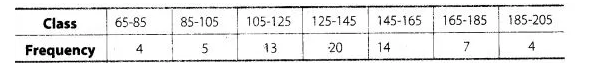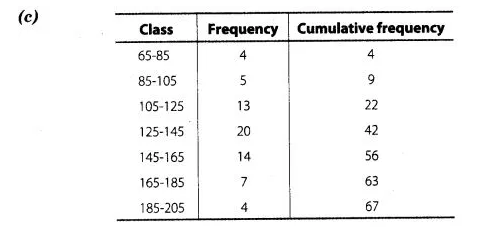# consider the data.

Question:

consider the data.The difference of the upper limit of the median class and the lower limit of the modal class is

(a) 0

(b) 19

(c) 20

(d) 38

Solution:Here, $\frac{N}{2}=\frac{67}{2}=33.5$ which lies in the interval $125-145$.

Hence, upper limit of median class is 145.

ere, we see that the highest frequency is 20 which lies in 125-145. Hence, the lower limit of modal class is 125.

Required difference = Upper limit of median class – Lower limit of modal class

= 145-125 = 20